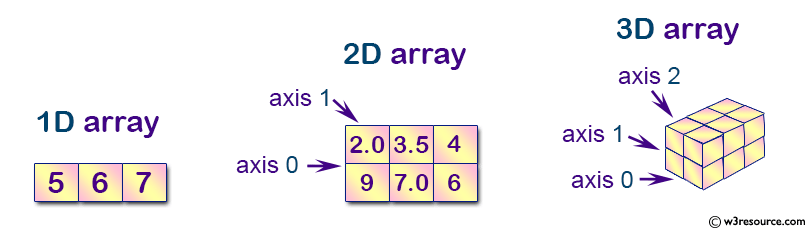# NumPy Tutorial

## What is NumPy?

NumPy is the fundamental package for scientific computing in Python. It is a Python library that provides a multidimensional array object, various derived objects (such as masked arrays and matrices), and an assortment of routines for fast operations on arrays, including mathematical, logical, shape manipulation, sorting, selecting, I/O, discrete Fourier transforms, basic linear algebra, basic statistical operations, random simulation and much more.

NumPy Basics

`import numpy as np`
Operator Description
np.array([1,2,3]) 1d array
np.array([(1,2,3),(4,5,6)]) 2d array
np.arange(start,stop,step) range array

Placeholders

Operator Description
np.linspace(0,2,9) Add evenly spaced values btw interval to array of length
np.zeros((1,2)) Create and array filled with zeros
np.ones((1,2)) Creates an array filled with ones
np.random.random((5,5)) Creates random array
np.empty((2,2)) Creates an empty array

Array

Syntax Description
array.shape Dimensions (Rows,Columns)
len(array) Length of Array
array.ndim Number of Array Dimensions
array.dtype Data Type
array.astype(type) Converts to Data Type
type(array) Type of ArrayCopying/Sorting

Operators Description
np.copy(array) Creates copy of array
other = array.copy() Creates deep copy of array
array.sort() Sorts an array
array.sort(axis=0) Sorts axis of array

Array Manipulation

Operator Description
np.append(a,b) Append items to array
np.insert(array, 1, 2, axis) Insert items into array at axis 0 or 1
np.resize((2,4)) Resize array to shape(2,4)
np.delete(array,1,axis) Deletes items from array

Combining Arrays

Operator Description
np.concatenate((a,b),axis=0) Concatenates 2 arrays, adds to end
np.vstack((a,b)) Stack array row-wise
np.hstack((a,b)) Stack array column wise

Splitting Arrays

Operator Description
numpy.split() Split an array into multiple sub-arrays.
np.array_split(array, 3) Split an array in sub-arrays of (nearly) identical size
numpy.hsplit(array, 3) Split the array horizontally at 3rd index

More

Operator Description
other = ndarray.flatten() Flattens a 2d array to 1d
array = np.transpose(other)
array.T
Transpose array
inverse = np.linalg.inv(matrix) Inverse of a given matrix

Mathematics

Operations

Operator Description
x + y
np.substract(x,y)
x - y
Subtraction
np.divide(x,y)
x / y
Division
np.multiply(x,y)
x @ y
Multiplication
np.sqrt(x) Square Root
np.sin(x) Element-wise sine
np.cos(x) Element-wise cosine
np.log(x) Element-wise natural log
np.dot(x,y) Dot product
np.roots([1,0,-4]) Roots of a given polynomial coefficients

Comparison

Operator Description
== Equal
!= Not equal
< Smaller than
> Greater than
<= Smaller than or equal
>= Greater than or equal
np.array_equal(x,y) Array-wise comparison

Basic Statistics

Operator Description
np.mean(array) Mean
np.median(array) Median
array.corrcoef() Correlation Coefficient
np.std(array) Standard Deviation

More

Operator Description
array.sum() Array-wise sum
array.min() Array-wise minimum value
array.max(axis=0) Maximum value of specified axis
array.cumsum(axis=0) Cumulative sum of specified axis

Slicing and Subsetting

Operator Description
array[i] 1d array at index i
array[i,j] 2d array at index[i][j]
array[i<4] Boolean Indexing, see Tricks
array[0:3] Select items of index 0, 1 and 2
array[0:2,1] Select items of rows 0 and 1 at column 1
array[:1] Select items of row 0 (equals array[0:1, :])
array[1:2, :] Select items of row 1
[comment]: <> ( array[1,...]
array[ : :-1] Reverses array

Importing/exporting

Syntax Description